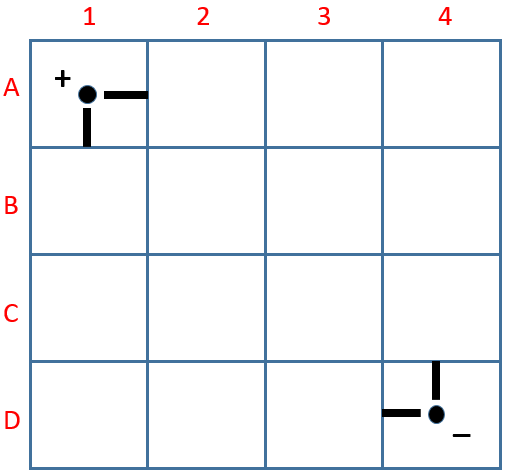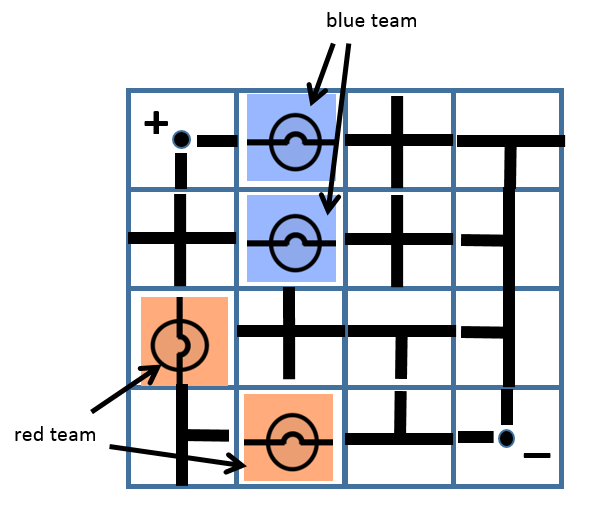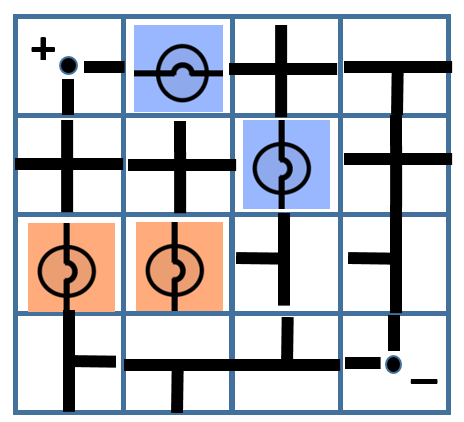# physics game on electricity

## DeLight Version 2I modified “DeLight”, the board game that I designed a few years back into a worksheet version (for small groups) as well as a powerpoint version (that teacher can facilitate as a class activity, pitting half the class against another).

The objectives of the game is to reinforce concepts related to D.C. Circuits such as:

1. Sum of potential difference (p.d.) across parallel branches of a circuit is the same.
$$E = V_1 + V_2 + V_3 +…$$
2. P.d. across a device is given by the ratio of resistance of device to total resistance multiplied by emf (potential divider rule)
$$V_1 = \dfrac{R_1}{R_{total}}\times E$$
3. Brightness of light bulb depends on electrical power
$$P = IV = \dfrac{V^2}{R} = I^2R$$
4. Current can bypass a device via a short-circuiting wire.

The worksheet and powerpoint slides contain a few examples that allow discussion on the above concepts based on some possible gameplay outcomes. For example, the following is a game where the blue team wins because the p.d. across each blue light bulb is twice that of the p.d. across each red light bulb.In the following scenario, the game ended in a draw. Students may not be able to see it immediately, but the blue light bulb with a vertical orientation is actually short-circuited by the vertical branch on its right.Feel free to use and/or modify the game to suit your own class needs.